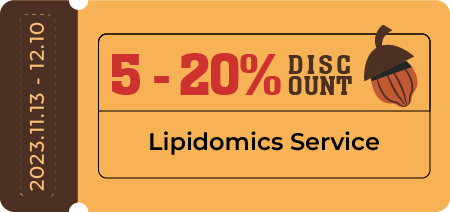# Untargeted Lipidomics Bioinformation Analysis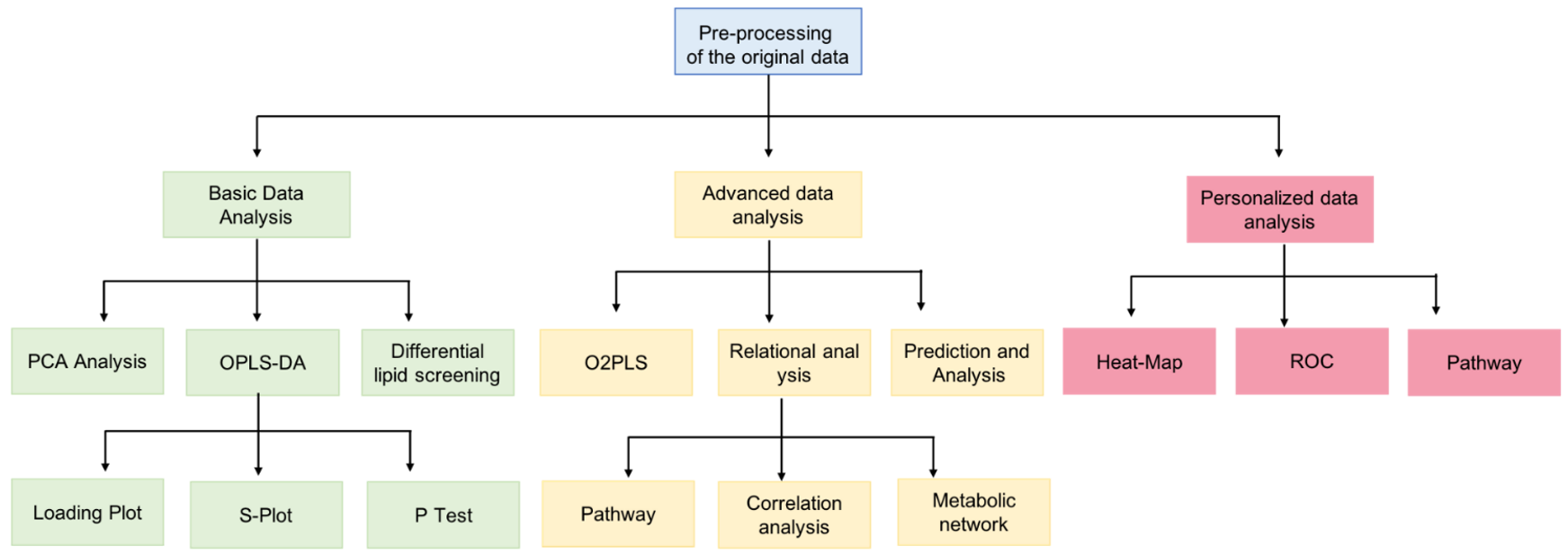### Data Preprocessing

1) Missing value processing:
- Missing value filtering
- Missing value filling: minimum filling, average/median filling, KNN (K-nearest neighbor) filling, BPCA (Bayesian PCA) filling, PPCA (probabilistic PCA) filling, Singular Value Decomposition (SVD)

2) Noise signal removal
- For a single ion peak, if the RSD is less than 0.3, the ion peak is qualified, otherwise it is removed.
- For the overall data, if RSD<0.3 and the proportion of peaks >60%, the overall data is qualified.

3) Sample normalization: improve the comparability between samples.

4) Data conversion: downstream analysis generally requires the data to be normal distribution or Gaussian distribution, so the data usually needs to be Log conversion or power conversion. Both of these can eliminate the suppression effect of the maximum value, and can adjust the distribution of the data.

### Data Quality Control

• Evaluate the following:
• TIC overlap of QC samples
• Proportion of peaks with CV<30% in QC samples
• The degree of aggregation of QC samples in PCA
• Relevance of QC samples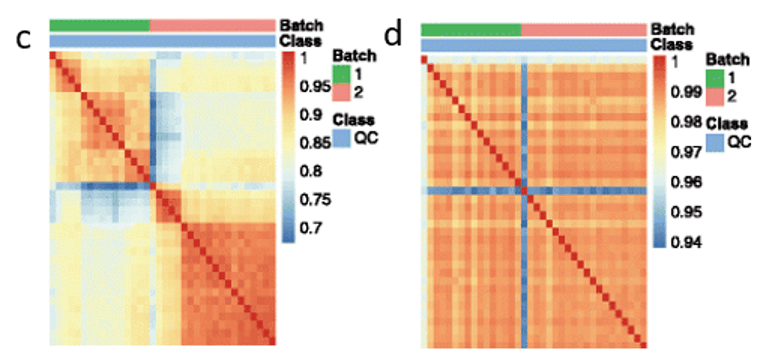### Statistical Analysis

1) Univariate analysis:
Analyze only one variable at a time, that is, one m/z, and check whether the m/z expression of different samples in different groups is different. Common methods include multiple analysis, t test, rank sum test, analysis of variance and so on.

2) Cluster analysis
According to specific indicators (variables), the samples under study are classified. Cluster analysis needs to set up a method to measure the similarity or dissimilarity between samples (usually Euclidean distance, correlation coefficient, etc.). Common clustering methods: systematic clustering (hierarchical clustering), K-means clustering, etc.

3) Multivariate analysis
- Principal component analysis (PCA)

PCA of samples can generally reflect the overall metabolic differences between samples in each group and the degree of variability between samples within the group.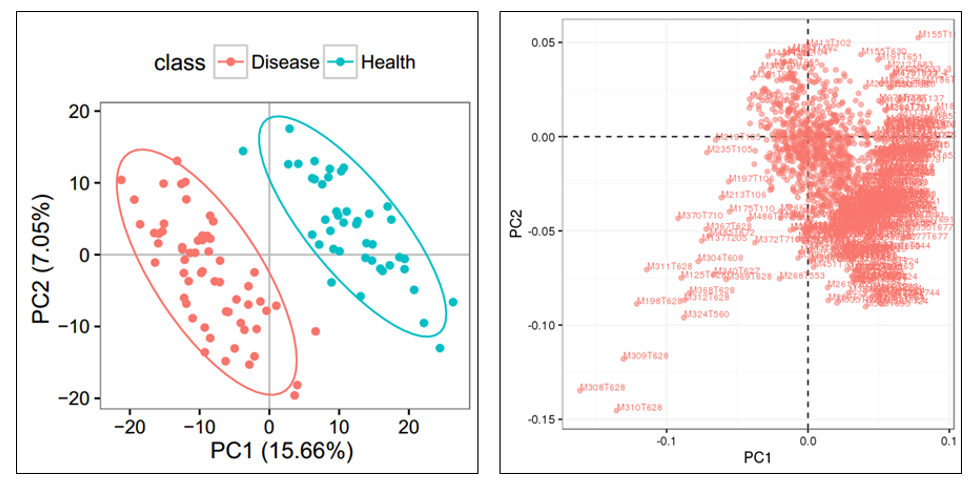- Partial least square (PLS) methods：PLSDA graph is similar to PCA

- OPLS-DA

To eliminate noise information that is not related to classification, and also to obtain related metabolite information that causes significant differences between the two groups, we use OPLS-DA to filter signals that are not related to model classification.

- Correlation analysis

The lipids identified by untargeted lipidomics or late-stage targeted lipidomics are correlated with phenotypes.

4) Construct a regression equation for prediction

5) Network analysis
- Enrichment analysis
- Pathway analysis

Topological analysis, which calculates a central location of metabolites in the network, is added to the pathway analysis and outputs the impact of the pathway in the overall network. The greater the importance, the more central the position may be in the whole pathway.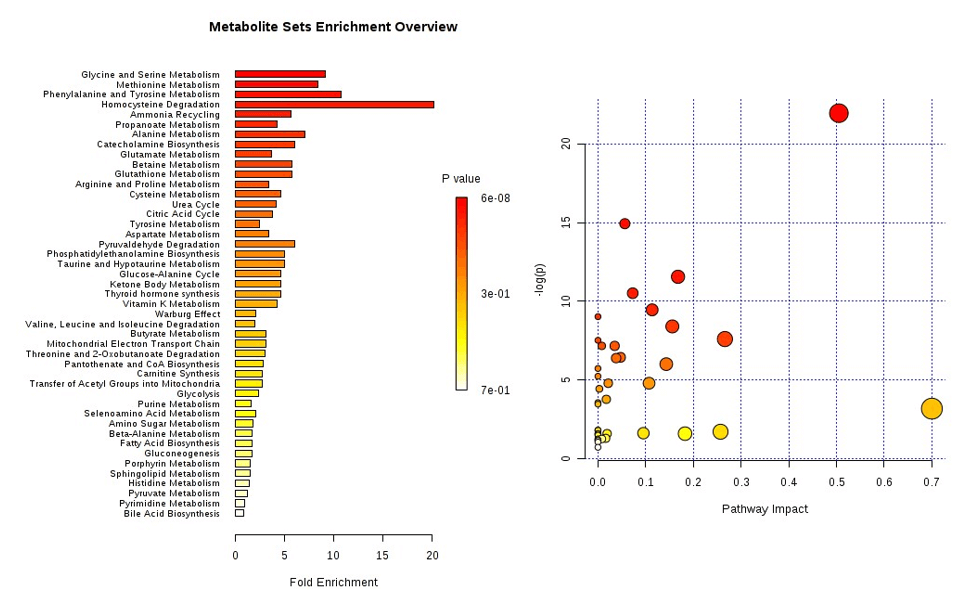* Our services can only be used for research purposes and Not for clinical use.

Services:

Resource:

Platform:

Online Inquiry

Services

Resource

• USA
• Tel: